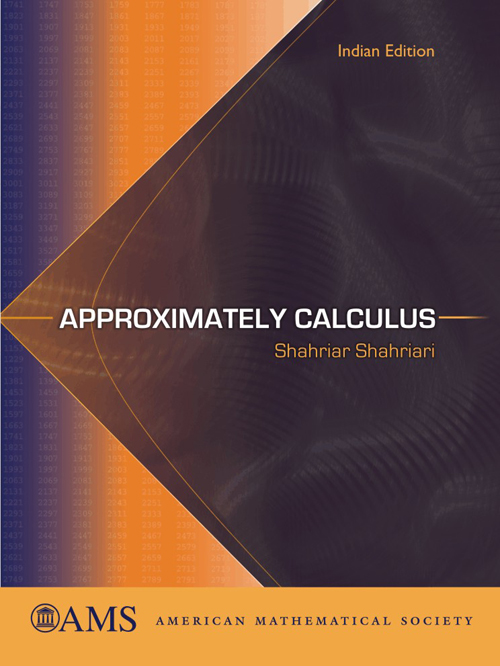Approximately Calculus
Shahriar Shahriari
Price
1525.00
ISBN
9780821887042
Language
English
Pages
312
Format
Paperback
Dimensions
180 x 240 mm
Year of Publishing
2012
Territorial Rights
Restricted
Imprint
American Mathematical Society
Catalogues

Is there always a prime number between n and 2n? Where, approximately, is the millionth prime? And just what does calculus have to do with answering either of these questions? It turns out that calculus has a lot to do with both questions, as this book can show you.

The theme of the book is approximations. Calculus is a powerful tool because it allows us to approximate complicated functions with simpler ones. Indeed, replacing a function locally with a linear–or higher order–approximation is at the heart of calculus. The real star of the book, though, is the task of approximating the number of primes up to a number x. This leads to the famous Prime Number Theorem--and to the answers to the two questions about primes.

While emphasizing the role of approximations in calculus, most major topics are addressed, such as derivatives, integrals, the Fundamental Theorem of Calculus, sequences, series, and so on. However, our particular point of view also leads us to many unusual topics: curvature, Padé approximations, public key cryptography, and an analysis of the logistic equation, to name a few.

The reader takes an active role in developing the material by solving problems. Most topics are broken down into a series of manageable problems, which guide you to an understanding of the important ideas. There is also ample exposition to fill in background material and to get you thinking appropriately about the concepts.

Approximately Calculus is intended for the reader who has already had an introduction to calculus, but wants to engage the concepts and ideas at a deeper level. It is suitable as a text for an honors or alternative second semester calculus course.

Shahriar Shahriari, Pomona College, Claremont, CA.

* Patterns and induction
* Divisibility
* Primes
* Derivatives and approximations of functions
* Antiderivatives and integration
* Distribution of primes
* Log, exponential, and the inverse trigonometric functions
* The mean value theorem and approximations
* Linearization topics
* Defining integrals, areas, and arc lengths
* Improper integrals and techniques of integration
* The prime number theorem
* Local approximation of functions and integral estimations
* Sequences and series
* Power series and Taylor series
* More on series
* Limits of functions
* Differential equations
* Logical arguments
* Hints for selected problems
* Bibliography
* Index

THE BOOKPOINT (INDIA) PVT. LTD.